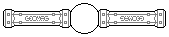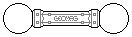Web textodigital.com
Construction of the rhombicosidodecahedron
The rhombicosidodecahedron has 60 vertices, 120 edges, and 62 faces (12 pentagons, 30 squares, and 20 triangles), and it doesn't require any auxiliary structure. So, it can be built using 60 balls, 120 rods, 12 pentagonal panels, and 30 squares. Triangles are not really necessary, but you can use 20 of them if you want.
First, we make the twelve pentagonal faces, in which task we will use 60 rods and all 60 balls. They can be nicely piled up, to save desk space and ease counting: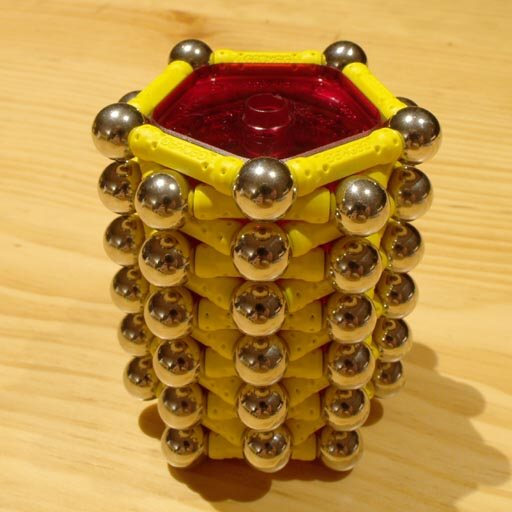Construction of the rhombicosidodecahedron, step 1
We add a rod-only triangle to each vertex of the first pentagon: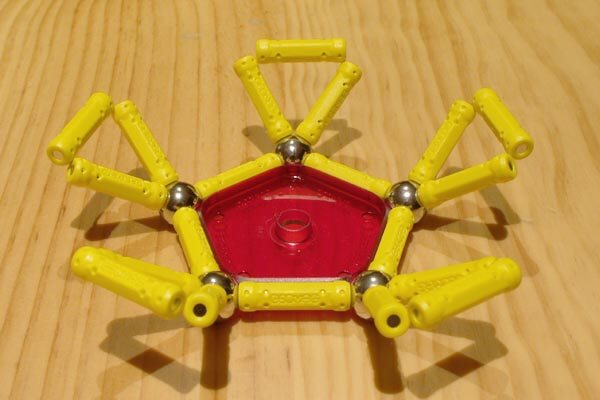Construction of the rhombicosidodecahedron, step 2
We join each pair of consecutive triangles with another pentagon, which simultaneously makes up five squares: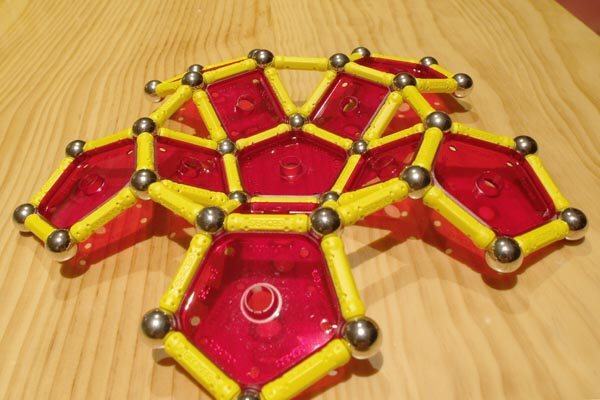Construction of the rhombicosidodecahedron, step 3
We lift the pentagons, and join each pair of them with more triangles (making up five squares, again). We also add triangles to the upper, free vertices of pentagons: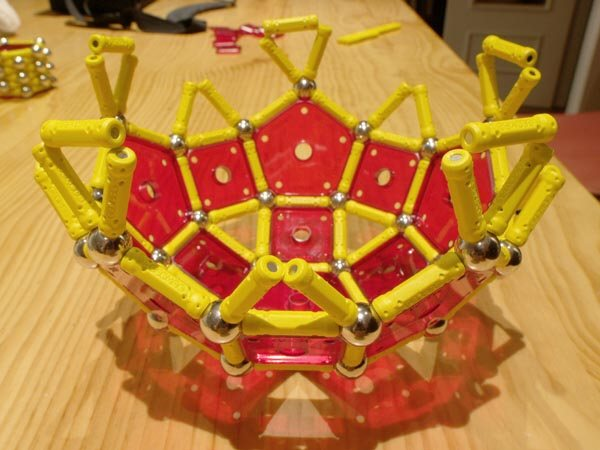Construction of the rhombicosidodecahedron, step 4
Now, we add five pentagons with a vertex pointing down. There are three free triangle vertices waiting for each, and the operation makes up ten squares. Five more triangles join the newly added pentagons, making up five more squares: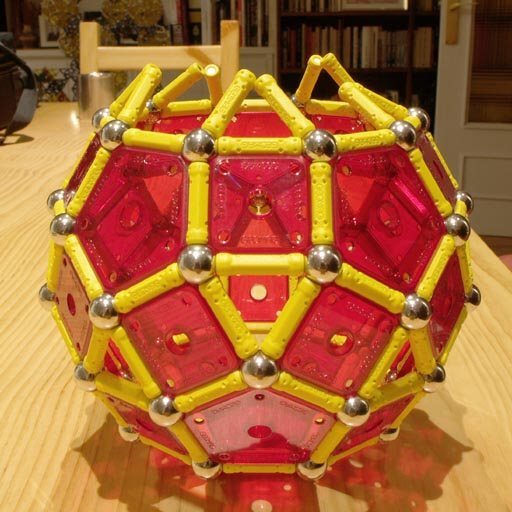Construction of the rhombicosidodecahedron, step 5
In the last step, not shown, we add the last pentagon on top, which, in turn, closes the last five squares.